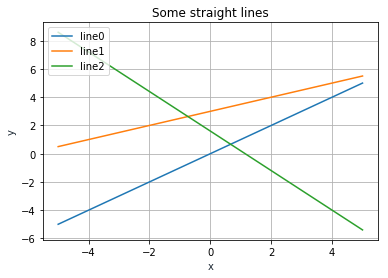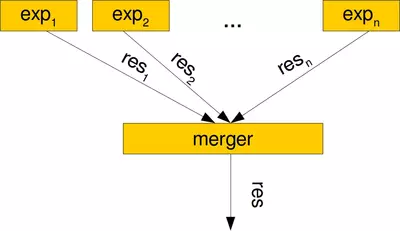# 10. Callable Instances of Classes

## The call method

There will be hardly any Python user who hasn't stumbled upon exceptions like 'dict' object is not callable or 'int' object is not callable. After a while they find out the reason. They used parentheses (round bracket) in situation where they shouldn't have done it. Expressions like f(x), gcd(x, y) or sin(3.4) are usually used for function calls.

The question is, why do we get the message 'dict' object is not callable if we write d('a'), if d is a dictionary? Why doesn't it say 'dict' object is not a function?

First of all, when we invoke a function in Python, we also say we 'call the function'. Secondly, there are objects in Python, which are 'called' like functions but are not functions strictly speaking. There are 'lambda functions', which are defined in a different way. It is also possible to define classes, where the instances are callable like 'regular' functions. This will be achieved by adding another magic method the __call__ method.Before we will come to the __call__ method, we have to know what a callable is. In general, a "callable" is an object that can be called like a function and behaves like one. All functions are also callables. Python provides a function with the name callable. With the help of this funciton we can determine whether an object is callable or not. The function callable returns a Boolean truth value which indicates whether the object passed as an argument can be called like a function or not. In addition to functions, we have already seen another form of callables: classes

def the_answer(question):
return 42


### OUTPUT:

the_answer:  True


The __call__ method can be used to turn the instances of the class into callables. Functions are callable objects. A callable object is an object which can be used and behaves like a function but might not be a function. By using the __call__ method it is possible to define classes in a way that the instances will be callable objects. The __call__ method is called, if the instance is called "like a function", i.e. using brackets. The following class definition is the simplest possible way to define a class with a __call__ method.

class FoodSupply:
def __call__(self):
return "spam"
foo = FoodSupply()
bar = FoodSupply()
print(foo(), bar())


### OUTPUT:

spam spam


The previous class example is extremely simple, but useless in practical terms. Whenever we create an instance of the class, we get a callable. These callables are always defining the same constant function. A function without any input and a constant output "spam". We'll now define a class which is slightly more useful. Let us slightly improve this example:

class FoodSupply:
def __init__(self, *incredients):
self.incredients = incredients
def __call__(self):
result = " ".join(self.incredients) + " plus delicious spam!"
return result
f = FoodSupply("fish", "rice")
f()


### OUTPUT:

'fish rice plus delicious spam!'

Let's create another function:

g = FoodSupply("vegetables")
g()


### OUTPUT:

'vegetables plus delicious spam!'

Now, we define a class with the name TriangleArea. This class has only one method, which is the __call__method. The __call__ method calculates the area of an arbitrary triangle, if the length of the three sides are given.

class TriangleArea:
def __call__(self, a, b, c):
p = (a + b + c) / 2
result = (p * (p - a) * (p - b) * (p - c)) ** 0.5
return result
area = TriangleArea()
print(area(3, 4, 5))


### OUTPUT:

6.0


This program returns 6.0. This class is not very exciting, even though we can create an arbitrary number of instances where each instance just executes an unaltered __call__ function of the TrianlgeClass. We cannot pass parameters to the instanciation and the __call__ of each instance returns the value of the area of the triangle. So each instance behaves like the area function.

After the two very didactic and not very practical examples, we want to demonstrate a more practical example with the following. We define a class that can be used to define linear equations:

class StraightLines():
def __init__(self, m, c):
self.slope = m
self.y_intercept = c
def __call__(self, x):
return self.slope * x + self.y_intercept
line = StraightLines(0.4, 3)
for x in range(-5, 6):
print(x, line(x))


### OUTPUT:

-5 1.0
-4 1.4
-3 1.7999999999999998
-2 2.2
-1 2.6
0 3.0
1 3.4
2 3.8
3 4.2
4 4.6
5 5.0


We will use this class now to create some straight lines and visualize them with matplotlib:

lines = []
lines.append(StraightLines(1, 0))
lines.append(StraightLines(0.5, 3))
lines.append(StraightLines(-1.4, 1.6))
import matplotlib.pyplot as plt
import numpy as np
X = np.linspace(-5,5,100)
for index, line in enumerate(lines):
line = np.vectorize(line)
plt.plot(X, line(X), label='line' + str(index))
plt.title('Some straight lines')
plt.xlabel('x', color='#1C2833')
plt.ylabel('y', color='#1C2833')
plt.legend(loc='upper left')
plt.grid()
plt.show()Our next example is also exciting. The class FuzzyTriangleArea defines a __call__ method which implements a fuzzy behaviour in the calculations of the area. The result should be correct with a likelihood of p, e.g. 0.8. If the result is not correct the result will be in a range of ± v %. e.g. 0.1.

import random
class FuzzyTriangleArea:
def __init__(self, p=0.8, v=0.1):
self.p, self.v = p, v
def __call__(self, a, b, c):
p = (a + b + c) / 2
result = (p * (p - a) * (p - b) * (p - c)) ** 0.5
if random.random() <= self.p:
return result
else:
return random.uniform(result-self.v,
result+self.v)
area1 = FuzzyTriangleArea()
area2 = FuzzyTriangleArea(0.5, 0.2)
for i in range(12):
print(f"{area1(3, 4, 5):4.3f}, {area2(3, 4, 5):4.2f}")


### OUTPUT:

5.993, 5.95
6.000, 6.00
6.000, 6.00
5.984, 5.91
6.000, 6.00
6.000, 6.00
6.000, 6.17
6.000, 6.13
6.000, 6.01
5.951, 6.00
6.000, 5.95
5.963, 6.00


Beware that this output differs with every call! We can see the in most cases we get the right value for the area but sometimes not.

We can create many different instances of the previous class. Each of these behaves like an area function, which returns a value for the area, which may or may not be correct, depending on the instantiation parameters p and v. We can see those instances as experts (expert functions) which return in most cases the correct answer, if we use p values close to 1. If the value v is close to zero, the error will be small, if at all. The next task would be merging such experts, let's call them exp1, exp2, ..., expn to get an improved result. We can perform a vote on the results, i.e. we will return the value which is most often occuring, the correct value. Alternatively, we can calculate the arithmetic mean. We will implement both possibilities in our class FuzzyTriangleArea:from random import uniform, random
from collections import Counter
class FuzzyTriangleArea:
def __init__(self, p=0.8, v=0.1):
self.p, self.v = p, v
def __call__(self, a, b, c):
p = (a + b + c) / 2
result = (p * (p - a) * (p - b) * (p - c)) ** 0.5
if random() <= self.p:
return result
else:
return uniform(result-self.v,
result+self.v)
class MergeExperts:
def __init__(self, mode, *experts):
self.mode, self.experts = mode, experts
def __call__(self, a, b, c):
results= [exp(a, b, c) for exp in self.experts]
if self.mode == "vote":
c = Counter(results)
return c.most_common(1)
elif self.mode == "mean":
return sum(results) / len(results)
rvalues = [(uniform(0.7, 0.9), uniform(0.05, 0.2)) for _ in range(20)]
experts = [FuzzyTriangleArea(p, v) for p, v in rvalues]
merger1 = MergeExperts("vote", *experts)
print(merger1(3, 4, 5))
merger2 = MergeExperts("mean", *experts)
print(merger2(3, 4, 5))


### OUTPUT:

6.0
6.0073039634137375


The following example defines a class with which we can create abitrary polynomial functions:

class Polynomial:
def __init__(self, *coefficients):
self.coefficients = coefficients[::-1]
def __call__(self, x):
res = 0
for index, coeff in enumerate(self.coefficients):
res += coeff * x** index
return res
# a constant function
p1 = Polynomial(42)
# a straight Line
p2 = Polynomial(0.75, 2)
# a third degree Polynomial
p3 = Polynomial(1, -0.5, 0.75, 2)
for i in range(1, 10):
print(i, p1(i), p2(i), p3(i))


### OUTPUT:

1 42 2.75 3.25
2 42 3.5 9.5
3 42 4.25 26.75
4 42 5.0 61.0
5 42 5.75 118.25
6 42 6.5 204.5
7 42 7.25 325.75
8 42 8.0 488.0
9 42 8.75 697.25


You will find further interesting examples of the __call__ function in our tutorial in the chapters Decorators and Memoization with Decorators. You may also consult our chapter on Polynomials.

Live Python training

Enjoying this page? We offer live Python training courses covering the content of this site.

Upcoming online Courses

Enrol here